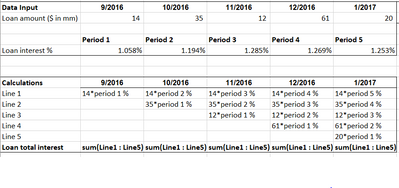# Cumulative interest calculationHi,

I need to calculate total loan interest for each month, and every loan was originated in different month. See below example, five loans were originated in different months and amount, and they have same interest % for each life period( i.e. loan x period 1 interest % is 1.058%, loan y period 1 interest % is also 1.058%)

Loan amount, origination month and interest % for each period are input. Any idea if I can just have "Loan total interest" line item in a module to do all the cal from line 1 to line 5?Thanks

Jay

Tagged: